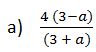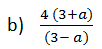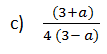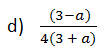# CMAT Quantitative Aptitude Practice Questions Set-1

CMAT Quantitative Aptitude Practice questions Set-1: This practice set has 10 questions with their answers covering topics from the areas of Quantitative Aptitude.

Nov 20, 2015 11:27 IST

CMAT Quantitative Aptitude Practice questions Set-1: This practice set has 10 questions with their answers covering topics from the areas of Quantitative Aptitude.

1. Three fair dices are thrown simultaneously. What is the probability that the sum is 5?

a) 1/36

b) 1/6

c) 1/54

d) 1/72

2. How many digits are used in writing the page numbers of the book from 1 to 500?

a) 1390

b) 1392

c) 1395

d) 1399

3. If log1227 = a, then log616 is:4. A Quadratic function f(x) attains a maximum of 3 at x = 1. The value of the function at x = 0 is 1. What is the value of f(x) at x = 10?

a) -180

b) -105

c) -110

d) -159

e) -119

5. A function f(x) satisfies f(1) = 3600 and f(1) + f(2) + f(3) +….+ f(n) = n2 (n) for all integers n > 1. What is the value of f(8).

a) 100

b) 80

c) 120

d) 90

6. Three containers contain alcohol to water in the ratios 3:5, 1:3 and 1:1 respectively. If the solutions of the three containers are mixed in the volume ratio of 1:2:3, then what is the ratio of alcohol to water in the final solution?

a) 5:9

b) 6:7

c) 12:19

d) 19:29

7. A thief has stolen a car from the point A at 8 AM and drives it away at 50 km/hr. The theft was discovered at 10 am and the owner starts chasing the thief at 10 am at the rate of 70 km/hr. At what time the owner will catch the thief?

a) 2 pm

b) 2:30 pm

c) 3 pm

d) 5 pm

8. Two train travel in opposite direction one at 30 kmph and the other at 42 kmph. A man sitting in the slower train passes the faster train in 6 s. what is the length of the faster train?

a) 120 m

b) 126 m

c) 140 m

d) 118 m

9. A alone can do a work in 24 days. B is 60 % more efficient than A & C is 50 % more efficient than B. All three of them working together will do the same work in how many days?

a) 3 days

b) 5 days

c) 7 days

d) 8 days

10. What are the last two digits of 7236?

(a) 36

(b) 56

(c) 76

(d) 96

 Questions Answers 1. A 2. B 3. A 4. D 5. A 6. D 7. C 8. A 9. B 10. D